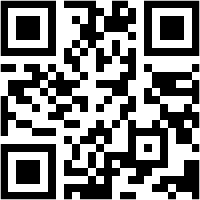Need Help? Chat with us

# Counta Function in Excel

## How to use Counta Function in Excel?

This tutorial will give you detailed information regarding the Microsoft Office Excel Counta Function. This short tutorial gives you information regarding:

## Special Offer for First Time Users

If you are the first time user and want to learn Microsoft office skills, then you can avail this special offer discount on your email.• What is Counta function in Excel?
• What happens if you combine Countif and Counta functions?
• The relation between Count, Counta, Countblank?
• Difference between Count and Counta function in excel?
• The formula to calculate Counta?
• Purpose of Counta?
• Where can you find Counta function?

? What is Counta Function in Excel?

Counta function is as simple as Count Function in Excel. Both the functions are straightforward functions.  Counta function counts the number of cells in a range that are non-empty. It is almost similar to count function. This function was first introduced in the Excel version 2000.

? What is the Purpose and Use of Counta Function in Excel?

The basic purpose of Counta function is to count cells that are not empty. This function is useful to us by letting us know the number of cells that include text, number, dates, and text strings etc. Even a space entered into the cell will be counted in COUNTA function. This function too is an inbuilt function in excel. It is categorized under Statistical Function.

? The Syntax or Formula of Counta Function in Excel:

The syntax used to count the Counta function is

=COUNTA(value1, [value2], …)

Where,

Value 1 represents the argument we want to count.

Value 2 represents the other 255 arguments that can be countered. (This is optional)

For Example: To count cells with the value in the range A1: A50 we use the formula as

=COUNTA(A1: A50)

It will represent the number of cells that are not empty in that specified range.

? Points to Remember:

Following are the points you must consider before you start working with Counta Function in Excel

• It does not count empty cells.
• The results might differ from the one we see.
• It counts the number of cells that contain not only numbers and dates but also string text, logical text, false text etc
• If you want to count numbers than you should use Count function rather than using Counta function.
• Countif and Countifs should be used only when we have to meet specific criteria.

? Where do you find the Counta Function?

You can find the Function in five different ways, which are as explained in detail below.

First: In the Autosum drop-down menu. Autosum drop down menu is at the home ribbon editing group with the Autosum name and sign ∑.

Second: Press “fx” function above the spreadsheet and below the ribbon. An Insert function dialog box will open. Select the function Counta.

Third: In the formula ribbon > Search for Autosum and click on the drop-down menu > Click on Counta.

Fourth: Go to the function library group > Click on More function > Click on statistical function > Select Counta Function.

Fifth: The Excel shortcut to open Insert function dialog box is Shift + F3. The insert function dialog box will pop up & Select Counta function.

? The relation between Count, Counta & Countblank:

The relationship between the three Count, Counta & Countblank function are explained below:

 Count Counta Countblank It only counts cell containing numbers. It counts cells containing everything except the empty cell. It counts only complete blank cells. Syntax =COUNT(range, values) Syntax =COUNTA(range, values) Syntax =COUNTBLANK(range)

? Difference between Count & Counta Function

The difference between the two Count & Counta are explained below:

 Count Function Counta Function Counts only numeric value. Counts numeric value along with logical text, text strings, false values Does do not count empty cells Counts empty cells that can be seen empty but it is actually not To count non-contagious range To count non-blank cell

? Conclusion:

While deleting the text from the cell we must see that we don’t use the backspace. We should use the delete button. This tutorial explained you about how COUNTA function was carried out and their relation with other functions as well.

Hope you like our tutorial for more cool and amazing trick of excel Like our Excel Superstar Facebook Page and subscribe to our Excel Superstar YouTube channel. Excel Superstar is the leading online training company, which provides Online Excel Course in Hindi. Connect with us and become an Excel Superstar.